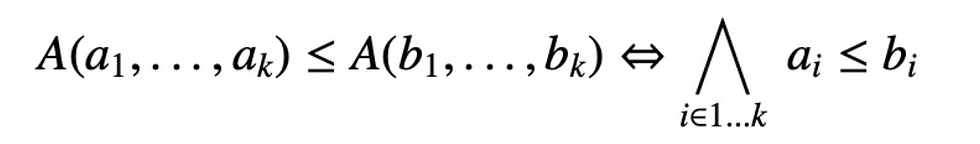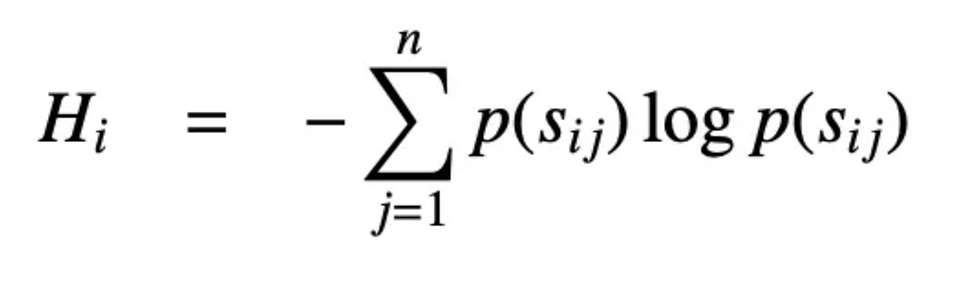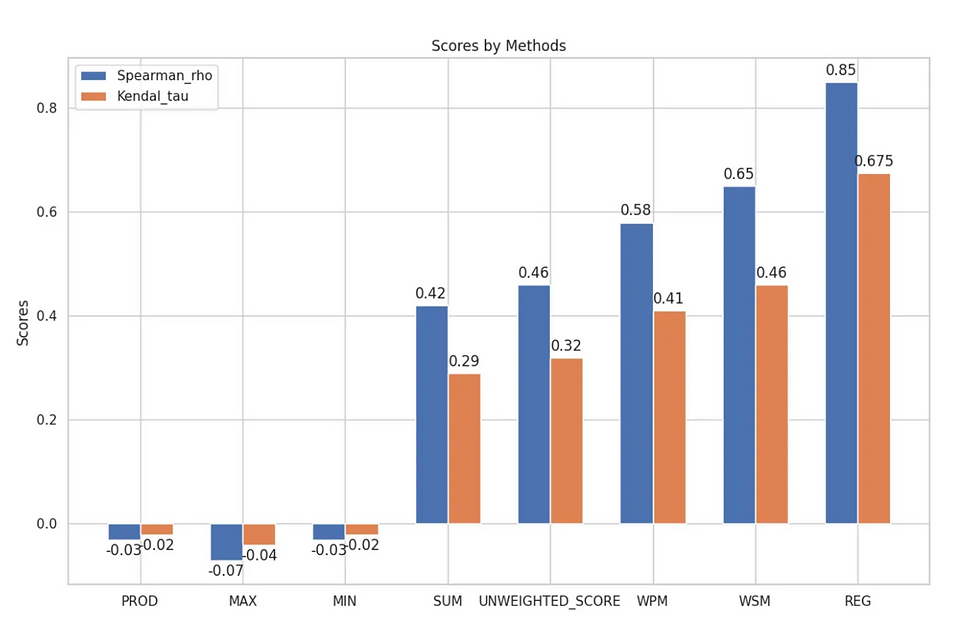top of page
Search

# Data Driven Aggregation ~Unsupervised Way

Updated: Aug 8Aggregation combines several independent variables to a dependent variable. The dependent variable should preserve properties of the independent variables, e.g., the ranking or relative distance of the independent variable tuples, and/or represent a latent ground truth that is a function of these independent variables. However, if ground truth data is not available for finding the aggregation model then what shall be the strategy to aggregate the information.

We will look at approach based on intrinsic properties of unlabeled training data, such as the cumulative probability distributions of the single independent variables and their mutual dependencies.

For assessing this against any aggregation approaches two perspectives are relevant:

(i) How well the aggregation output represents properties of the input tuples.

(ii) How well can aggregated output predict a latent ground truth.

We will be evaluating aggregation approaches from both perspectives at end. We will use data sets for assessing supervised regression approaches that contain explicit ground truth labels. However, the ground truth is not used for deriving the aggregation models, but it allows for the assessment from a perspective.

## Defining Aggregation:

Aggregation maps several input variables to a single output variable. We assume that the number of input variables is fixed, say k. W.l.o.g we also assume that all input and the output variables are of the unit interval [0, 1]. Formally, a function that maps the (k-dimensional) unit cube onto the unit intervalis called an aggregation function if it satisfies the following properties:

Monotonicity:Boundary conditions:A special case of aggregation is the aggregation of a singleton, i.e., the unary operator A : [0, 1] |→ [0, 1], that usually used to get a score or index for a single variable.

## Basic Aggregation:

Basic aggregation functions can be classified into three main classes with specific behavior and semantics. These classes are described below

Conjunctive aggregation :

It combines values like a logical AND operator, i.e., the result of aggregation can be large if all values are large. The basic rule is that the output of aggregation is bounded from above by the lowest input. If one of the inputs equals 1, then the output of aggregation is equal to the degree of our satisfaction with the other input variables. If any input is 0, then the output must be 0 as well. For example, if to obtain a driving license one has to pass both theory and driving tests, no matter how well one does in the theory test, it does not compensate for failing the driving test. From the set of basic conjunctive aggregations, we compare minimum MIN and product PROD in our evaluation.

Disjunctive aggregation:

It combine values like a logical OR operator, i.e., the result of aggregation can be large if at least one of the values is large. The basic rule is that the output of aggregation is bounded from below by the highest input. In contrast to conjunctive, satisfaction of any of the input variables is enough by itself. For example, when you come home both an open door and the alarm are indicators of a burglary, and either one is sufficient to raise suspicion. If both happen at the same time, they can reinforce each other, and our suspicion might become even stronger than the suspicion caused by any of these indicators by itself. From the set of basic disjunctive aggregations, we compare maximum MAX and sum SUM in our evaluation.

Averaging aggregation:

It is also known as compensative and compromising aggregation, a high (low) value of one input variable can be compensated by a low (high) value for another one and the result will be something in between. The basic rule is that the output of aggregation is lower than the highest input and larger than the lowest input. Note that the aggregation function MIN (MAX) is at the same time conjunctive (disjunctive) and the extreme cases of an averaging aggregation.

## Data-Driven Aggregation:

Data-driven approaches need tuples of variable values to define the aggregation function. Unsupervised approaches only require tuples of input variable values while supervised approaches require tuples of input and out variable values. Once the aggregation function is learned, it can be applied to all possible input variable tuples.

Regression:

it’s widely used in research and applications are instances of weighted arithmetic mean. Weights usually indicate the importance of the input variables and can be set by experts or calculated from raw data . In case both input and response variables are known, weights can be adjusted to fit the raw data by solving an optimization problem that minimizes an error. One basic way to solve this problem is to use linear regression.

Weighted quality scoring (WQS) :

It’s a fully automated unsupervised approach based on the weighted product model for aggregation. Based on input tuples, it normalizes the input variables to be not correlated negatively, i.e., they have the same direction. It then calculates weights that account the variation of values of a single input variable and for the interdependence between all input variables.

## Relatable Concepts with Aggregation :

Fusion:

The integration of information from several sources is known as data fusion. Different fusion techniques have been used in areas such as statistics, machine learning, sensor networks, robotics, and computer vision. The goal of fusion is to combine data from multiple sources to produce information better than would have been possible by using a single source. Improved information could mean less expensive, more accurate, or more relevant information. The goal of data aggregation is to combine data from multiple variables by reducing and abstracting it into a single one. In this sense, data aggregation is a subset of data fusion.

Machine learning :

Machine learning (ML) defines models that map input to output variables using example values of the variables. Based on the learning approach, we distinguish unsupervised ML, where models are learned solely based on tuples of input variables, from supervised ML, where models are learned based on tuples of input and output variables, and from feedback ML, where models are learned incrementally based on feedback on suggested output values. The aggregation techniques WSM and WPM are examples of unsupervised ML; the aggregation technique REG is an example of supervised ML. The basic aggregation techniques are not ML examples.

## Dimensionality Reduction

Aggregation is somewhat related to but still different from dimensionality reduction i.e., the embedding of elements of a high-dimensional vector space in a lower-dimensional space. Approaches to dimensionality reduction include principal component analysis (PCA) , stochastic neighbor embedding (SNE) , and its t-distributed variant t-SNE . One could think that the special case of reducing a multi-dimensional vector space (of metrics) to just one dimension (of a quality score) is a problem equivalent to aggregation. However, dimensionality reduction only aims at preserving the neighborhood of vectors of the original space in the reduced space. In contrast to that, aggregation assumes a latent total order in the measured artifacts related to the orders in each (metric) dimension that is to be aggregated. The aim is to define a totally ordered score order based on the partial orders induced by the (metric) dimensions that matches the latent order of the measured artifacts.

## Now let’s discuss the Framework:

We will be exploring on basic aggregation to data driven approaches like Regression and Weighted Quality Scoring techniques. This particular framework executes and apply each methods and finally evaluates on them.

Aggregation approaches

Below we formally define the aggregation approaches selected for comparison PROD, MIN, MAX, and SUM apply the respective basic aggregation functions:REG calculates a weighted arithmetic mean of the input values, WSM and WPM calculate a weighted sum and product, resp., of the normalized input values:For the ML approaches REG, WSM, and WPM, the weights wi and the normalization functions si , where i = 1 . . . k, are learned from data. Let each input variable x1, . . . , xk and response variable y have n instances; we denote their j-th values by x1 j , . . . , xk j, and yj , resp.

For REG, weights are learned using the least squares approach to minimize the sum of error squares in the training data:For WSM and WPM, scores and weights are learned as follows. Let 1 be the indicator function with 1(cond) = 1 if cond and 0, otherwise, and let cor be Pearson’s coefficient of correlation.Si(xij) score are normalized scores. This metrics normalization methodology used is the so-called probability integral transform, which is widely used in multivariate data analysis to transform random variables in order to study their joint distribution.

For the entropy based weights w ent i , we define Hi , the entropy of the normalized variable xi . Let si j = si(xi j).where p(si j) is the empirical frequency of si j. For the dependency based weights w dep i , we define ρi , the dependency of aggregation on each variable xi . Therefore, let Ri and R be two rankings of the data points (x1 j , . . . , xk j) ascending in ri and r, resp., where:Then ρi is the absolute value of Spearman’s rank order correlation ρ of these two rankings:These equations gives self-contained definition of how the learning of the normalization functions and the weights work for WSM and WPM.

## Evaluation measures

The choice of evaluation measures depends on the purpose of aggregation and on the availability of a ground truth. We evaluate aggregation approaches taking into account both external (ground truth) and internal (raw data) information. For external evaluation, we study how well aggregation output agrees with a ground truth. Therefore, we measure predictive power and similarity. For internal evaluation, we study how well aggregation output represents the properties of the input variables. Therefore, we measure consensus.

Predictive power:

We measure the correlation between aggregation output and ground truth to assess the ordering, relative spacing, and possible functional dependency. We use Spearman’s rho as a correlation coefficient to measure the pairwise degree of association between the values, i.e., how well a monotonic function describes the relationship. High values indicate a strong predictive power.

Similarity:

Moreover, we rank the data points according to the aggregation output and to the ground truth in acceding order. We measure a distance between the two rankings based on the Kendall’s tau distance to assess the number of pairwise disagreements between two rankings . It corresponds to the number of transpositions that bubble sort requires to turn one ranking into the other. Low values indicate a high similarity. In our metrics score calculation we have used Kendall’s tau correlation coef which is slightly modified version of Kendall’s tau distance. Here higher the value means better agreements.Consensus:

We rank the data points according to aggregation output and according to each input variable in acceding order. We use the Kemeny distance, i.e., the sum of the k Kendall tau distances between aggregation output and input rankings, to assess a consensus between the aggregation output and the input variables. Low values indicate a strong consensus.

## Experiment

Application of defined approaches are suitable for problem where you would like to have order among the data points in accordance to approximate the ground or latent truth.

We took air quality data to evaluate where we have response variable too so that we can do both internal as well as external evaluations.

Sample data :Derived Weights from Unsupervised Learning:By studying on this data we observe Dew point temperature have the highest of

contribution.

MetricsWhile looking at metrics we simply saw that WSM outperforms every other unsupervised aggregation while REG top’s the list and that’s obvious as it’s is supervised in nature.

## Conclusion

We discussed a weighted metrics aggregation approach . We defined probabilistic scores based on input distributions and weights as a degree of disorder and dependency. We aggregated scores using the weighted product of normalized metrics. As such, the aggregation is agnostic to the actual metrics and the quality assessment goal and could be theoretically applied in any quality scoring and ranking context where multi-modal quality or ranking criteria need to be condensed to a single score. Its benefit is that models can be constructed using unsupervised learning when training data with a ground truth is lacking.